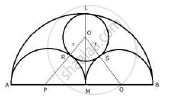Share

Ab is a Line Segment and M is Its Mid – Point Three Semi Circles Are Drawn with Am, Mb and Ab as Diameter on Same Side of Line Ab.A Circle with Radius R Unit is Drawn So Three Semi Circles Ab = 6 × R - ICSE Class 10 - Mathematics

ConceptArc and Chord Properties - Angle in a Semi-circle is a Right Angle

Question

AB is a line segment and M is its mid – point three semi circles are drawn with AM, MB and AB as diameter on the same side of the line AB. A circle with radius r unit is drawn so  that it  touches all the three semi circles show that : AB = 6 × r

Solution'

Let O, P and Q be the centers of the circle and semicircles.
Join OP and OQ.
OR = OS = r

and AP = PM = MQ = QB =(AB)/4

Now, OP = OR + RP = r + (AB)/4 (since PM=RP=radii of same circle)

Similarly, OQ = OS + SQ = r + (AB)/4

OM = LM -; OL =(AB)/2-r

Now in Rt. ΔOPM,
OP^2 = PM^2  + OM^2

⇒ (r = (AB)/4)^2 = ((AB)/4)^2 +((AB)/2 - r)^2

⇒ r^2 + "AB"^2/16 + "rAB"/2 = "AB"^2/16 + "AB"^2/4 + r^2 - rAB

⇒ "rAB"/2 = "AB"^2/4 - rAB

⇒ "AB"^2/4 =  "rAB"/2 + rAB

⇒  "AB"^2/4 = "3rAB"/2

⇒ "AB"/4 = 3/2 r

⇒ AB = 3/2 rxx 4 = 6  r

Hence AB = 6 × r

Is there an error in this question or solution?

Video TutorialsVIEW ALL 

Solution Ab is a Line Segment and M is Its Mid – Point Three Semi Circles Are Drawn with Am, Mb and Ab as Diameter on Same Side of Line Ab.A Circle with Radius R Unit is Drawn So Three Semi Circles Ab = 6 × R Concept: Arc and Chord Properties - Angle in a Semi-circle is a Right Angle.
S